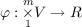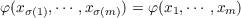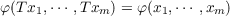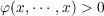#### Vol. 31, No. 1, 1969

 Download this articleFor screen For printingRecent Issues Vol. 325: 1  2 Vol. 324: 1  2 Vol. 323: 1  2 Vol. 322: 1  2 Vol. 321: 1  2 Vol. 320: 1  2 Vol. 319: 1  2 Vol. 318: 1  2Online Archive Volume: Issue:The Journal Subscriptions Editorial Board Officers Contacts Submission Guidelines Submission Form Policies for Authors ISSN: 1945-5844 (e-only) ISSN: 0030-8730 (print) Special Issues Author Index To Appear Other MSP Journals
Symmetric positive definite multilinear functionals with a given automorphism

### Marvin David Marcus and Stephen J. Pierce

Vol. 31 (1969), No. 1, 119–132
##### Abstract

Let V be an n-dimensional vector space over the real numbers R and let φ be a multilinear functional,(1)

i.e., φ(x1,,xm) is linear in each xj separately, j = 1,,m. Let H be a subgroup of the symmetric group Sm. Then φ is said to be symmetric with respect to H if(2)

for all σ H and all xj V,j = 1,,m. (In general, the range of φ may be a subset of some vector space over R.) Let T : V V be a linear transformation. Then T is an automorphism with respect to φ if(3)

for all xi V,i = 1,,m. It is easy to verify that the set A(H,T) of all φ which are symmetric with respect to H and which satisfy (3) constitutes a subspace of the space of all multilinear functionals symmetric with respect to H. We denote this latter set by Mm(V,H,R).

We shall say that φ is positive definite if(4)

for all nonzero x in V , and we shall denote the set of all positive definite φ in A(H,T) by P(H,T). It can be readily verified that P(H,T) is a convex cone in U(H,T).

Our main results follow.

Primary: 15.60
##### Milestones